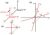# Numerical Methods And Simulation Techniques For Scientists And Engineers

Free!

language English Optional, paid
Category:

The course contains very important aspects of modern day course curriculum, namely, numerical methods and simulation techniques that are going to be of utmost importance to both undergraduate and graduate level. Most of the real life problems are unsolvable using known analytic techniques, thus depending on numerical methods is imperative. The course introduces basic numerical methods and the key simulation techniques that are going to be useful to academia and industry alike. Even if the software packages, such as Mathematica, Matlab etc are available for most of the numeric computations, yet one should be aware of the techniques that are inbuilt into the softwares.

COURSE LAYOUT

Week 1: Introduction to Numerical analysis, Importance of error and their calculations, Examples
Week 2: Root Finding Method of non-linear equations, Bisection Method, Newton Raphson Method,
Secant method, Regula- Falsi method, Practical examples.
Week 3: Curve fitting method, linear and non-linear fitting, Linear interpolation, Lagrange interpolation
method, Newton Interpolation formula, Practical examples.
Week 4: Numerical differentiation, central difference methods, higher order derivatives, errors, practical examples.
Week 5: Numerical integration, Simpson’s 1/3 rd rule, Simpson’s 3/8 th rule, local and global error analysis
,practical examples.
Week 6: Eigenvalue problems, Heun’s method, Euler’s method, Runge Kutta Method, Gerschgorin disc theorem ,
Jacobi method, Practical examples
Week 7: Simulation Techniques, Random numbers, Monte Carlo Method, Importance Sampling, Metropolis Algorithm,
Heat- bath algorithm, practical Examples
Week 8: Molecular dynamics, interaction and forces in molecular systems, MD and Verlet algorithm, correlations,
practical examples.

## User Reviews

0.0 out of 5
0
0
0
0
0

There are no reviews yet.

×